(ii) Unit Circle Trigonometric Functions
The connection of the trigonometric functions to the unit circle is commonly made by use of what is called "the wrapping function".

Consider a central angle on a unit circle in the cartesian plane with one ray the positive horizontal axis. Use its radian measure, $t$, as the length of the arc measured from the point $(1,0)$.
If $t >0$ the central angle is measured counterclockwise from ray of the positive horizontal axis.
If $t <0$ the central angle is measured clockwise from ray of the positive horizontal axis.
The endpoint of this arc on the unit circle, $P(t) = (x(t), y(t)$, is a function of the number $t$ and the angle measured by $t$.
This function $P: t \to P(t)= (x(t),y(t))$ is called the wrapping function. The wrapping function is visualized by a mapping diagram from a number line to the unit circle in a cartesian plane.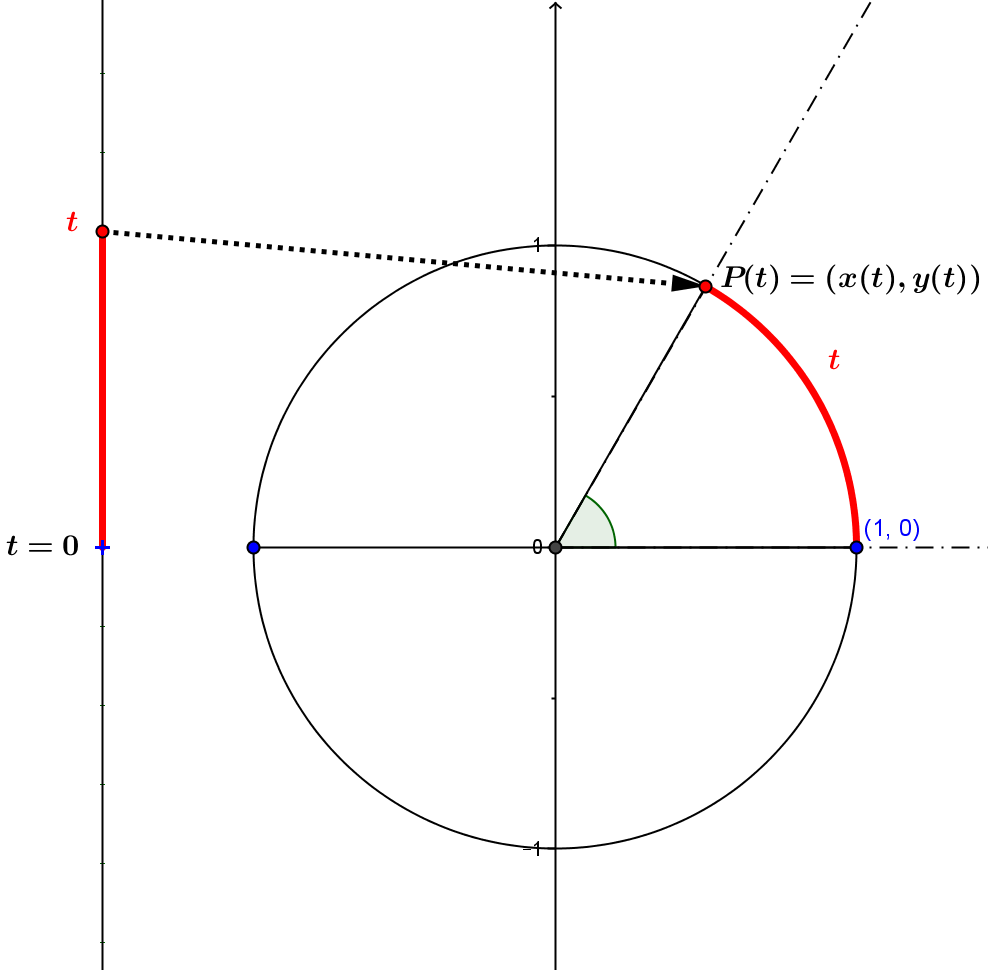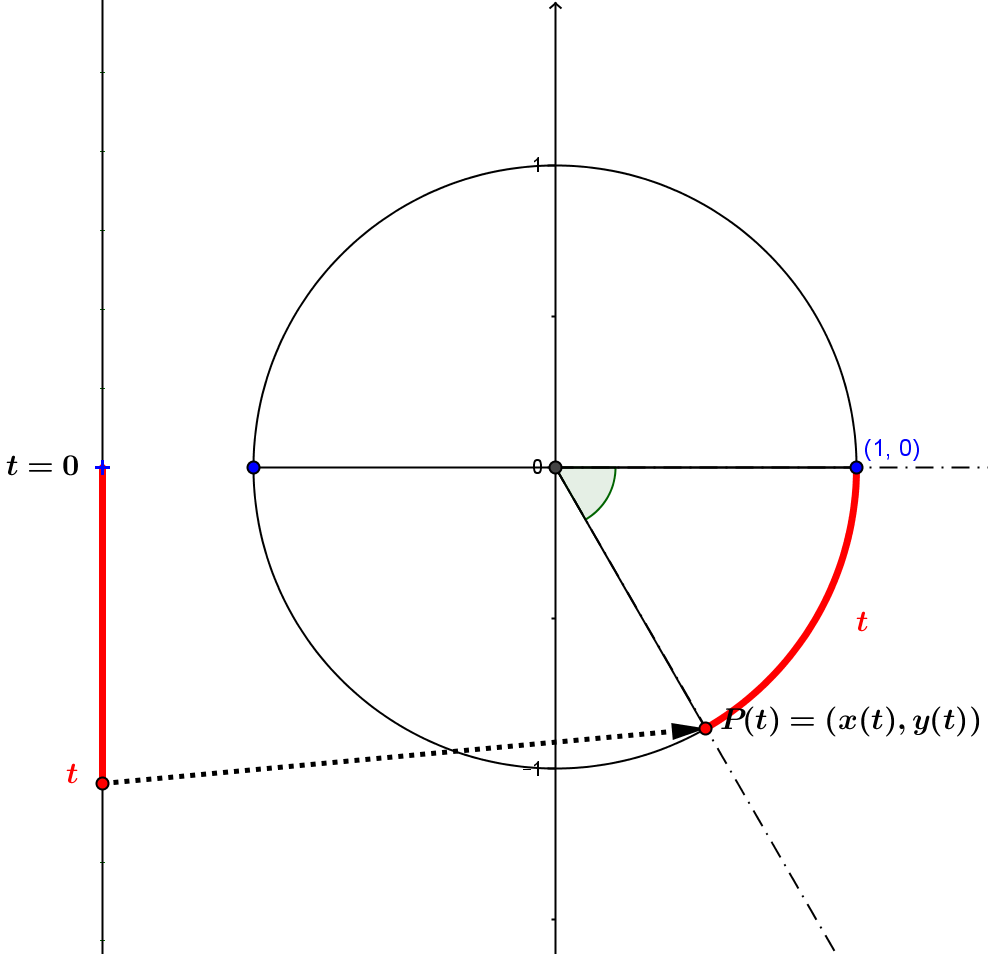After determining the point on the unit circle $P(t)$ by the wrapping function, a projection function based on the coordinates of $P(t)$ onto a line in that plane is used to determine the sine, cosine, and tangent function values as follows:

sine: Project $P(t)$ orthogonally onto the vertical axis to the point $(0,y(t))$ (applying $pr_y$ as the projection function) to obtain $pr_y(P(t)) =y(t) = \sin(t)$.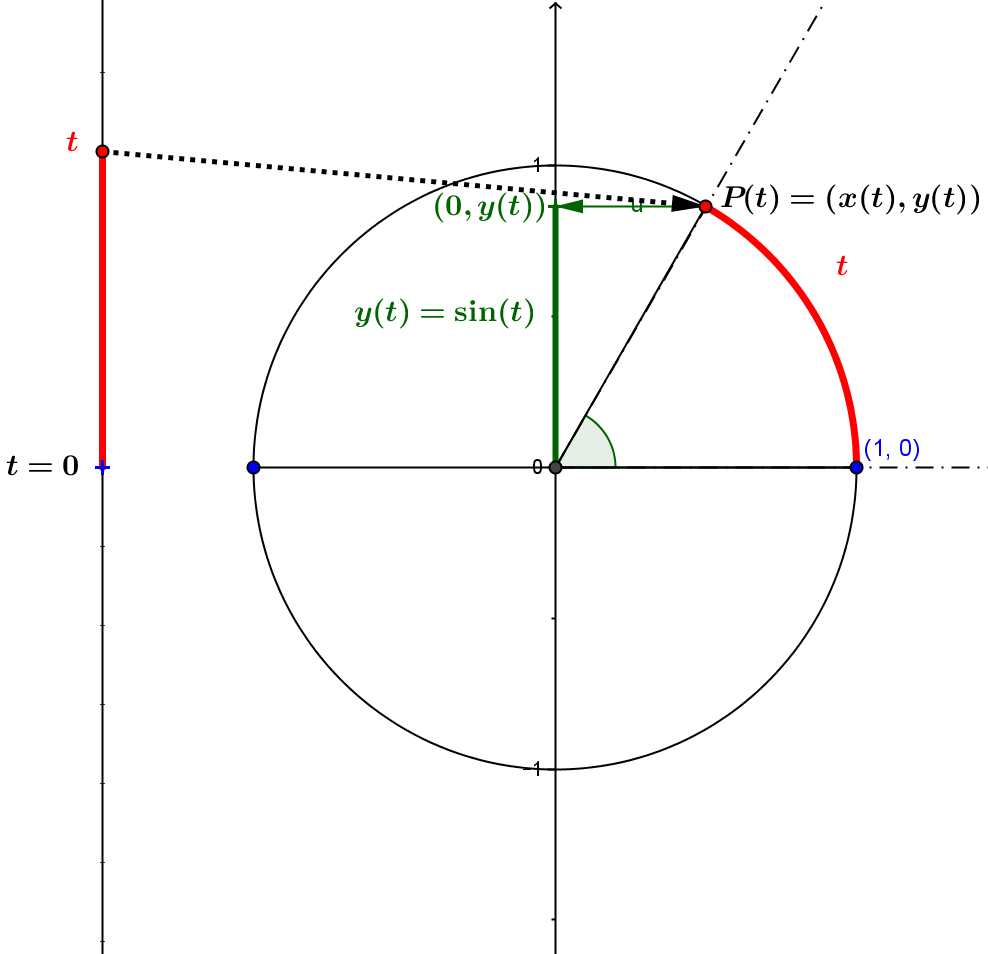cosine: Project $P(t)$ orthogonally onto the horizontal axis to the point $(x(t),0)$ (applying $pr_x$ as the projection function) to obtain $pr_x(P(t)) =x(t) = \cos(t)$.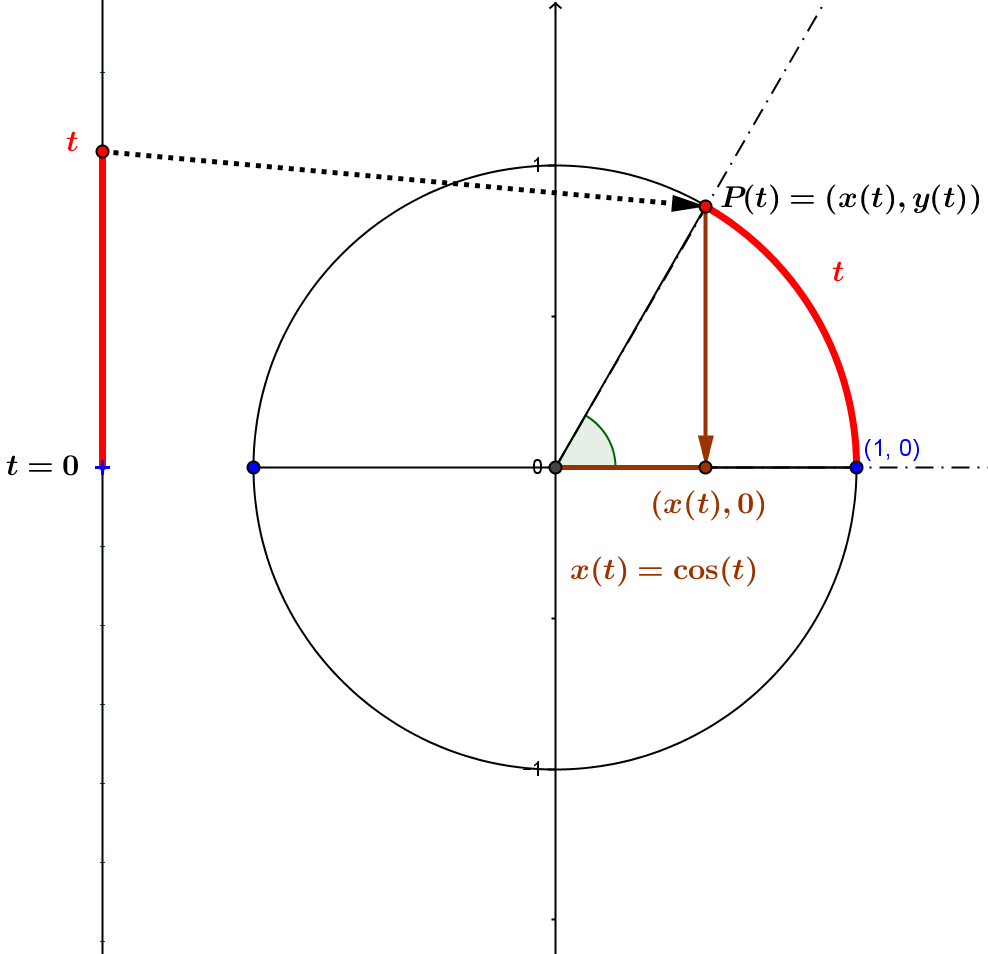tangent: Project $P(t)$ from the point $(0,0)$ to the vertical line $x=1$ to the point $(1, m(t))$ (applying $pr_{(0,0), (x=1)}$ as the projection function) to obtain $pr_{(0,0), (x=1)}(P(t)) = m(t) =\frac {y(t)}{x(t)}= \tan(t)$.
Notes: The tangent function is not defined when $x(t) =0$. Thus the domain for the tangent function is $Dom_{tan}=\{ t \in \mathbb R : t \ne (2k+1)\frac\pi 2, k \in \mathbb Z\}$
For $t \in Dom_{tan}$, we have the identity, $tan(t)=\frac{sin(t)}{cos(t)}$.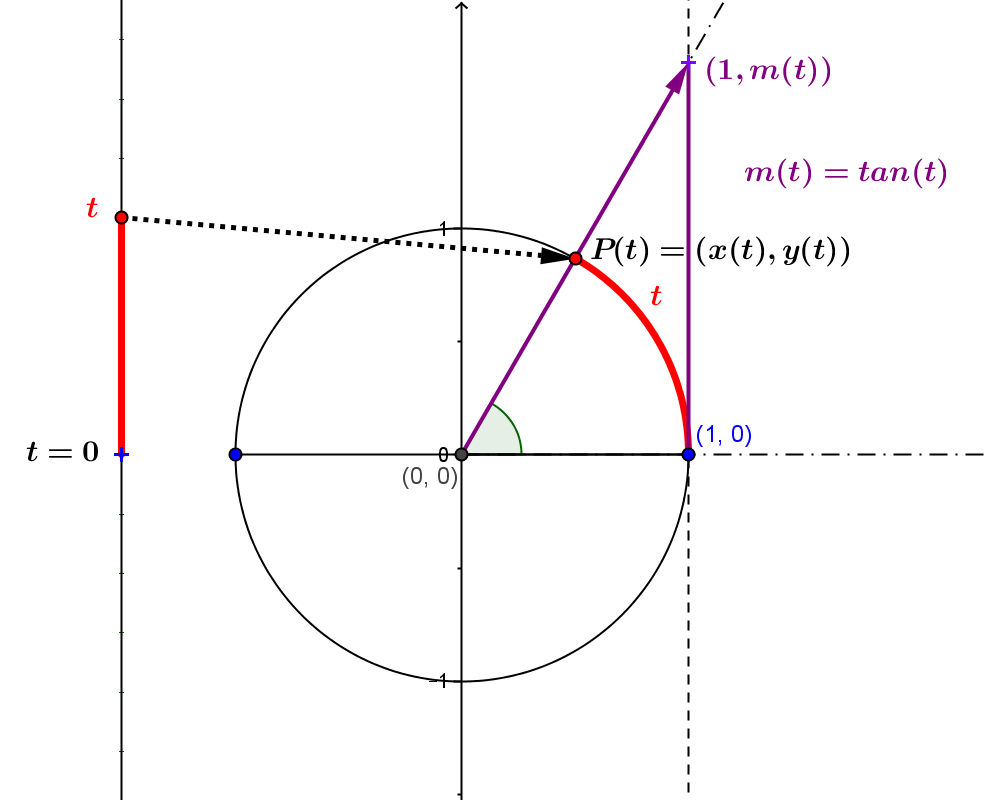cotangent: Project $P(t)$ from the point $(0,0)$ to the horizontal line $y=1$ to the point $(m_c(t),1)$ (applying $pr_{(0,0), (y=1)}$ as the projection function) to obtain $pr_{(0,0), (y=1)}(P(t)) = m_c(t) =\frac {x(t)}{y(t)}= \cot(t)$.
Notes: The cotangent function is not defined when $y(t) =0$. Thus the domain for the tangent function is $Dom_{cot}=\{ t \in \mathbb R : t \ne k\pi , k \in \mathbb Z\}$
For $t \in Dom_{cot}$, we have the identity, $cot(t)=\frac{cos(t)}{sin(t)}$.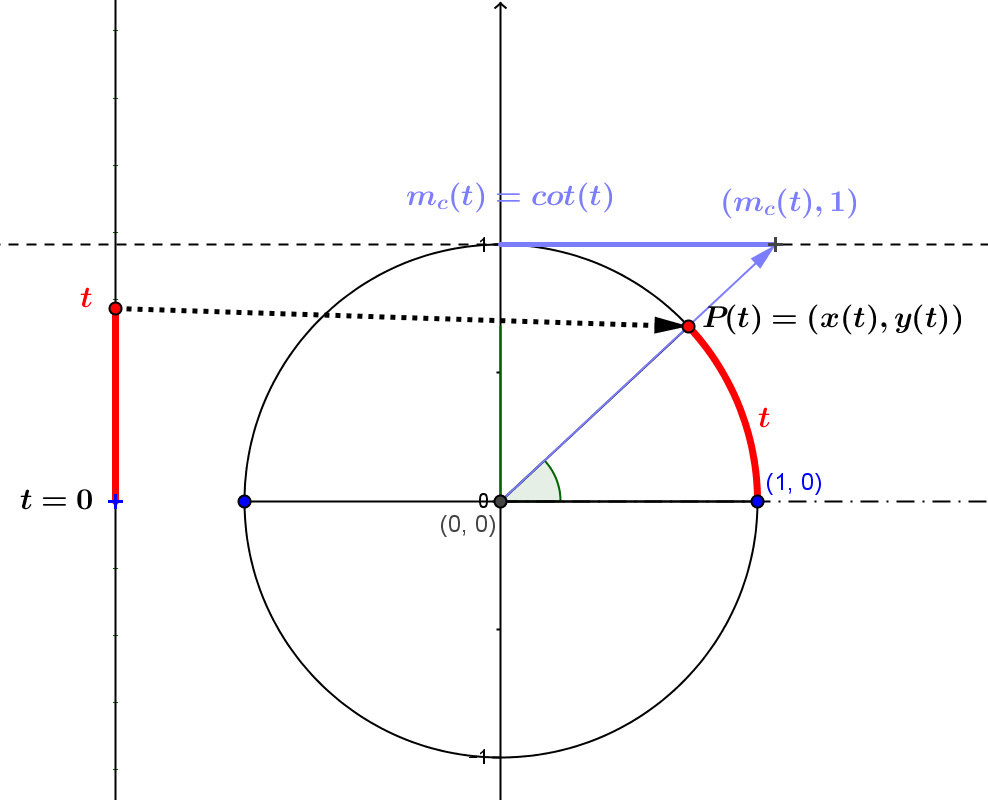Unit Circle Definition of Sine, Cosine, and Tangent: GeoGebra Mapping Diagrams